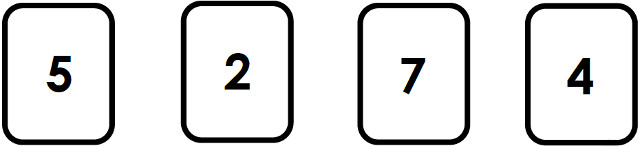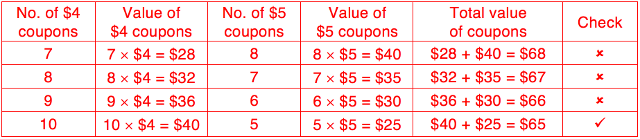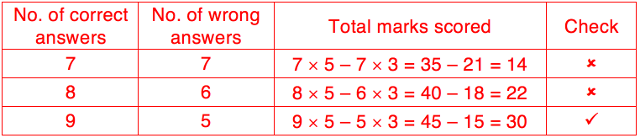The skills children pick up in math are indispensable; they can be applied to other academic subjects and to solve real-world problems in their daily lives and future work.

The Singapore Math curriculum focuses on problem-solving. Through problem-solving, children develop thinking skills such as creative thinking and critical thinking.

When children analyse math problems, they notice patterns, learn to generalise, form new ideas and activate their creative thinking. Children become critical thinkers when they are able to select the best strategy out of multiple methods to solve word problems.Singapore Math Heuristics

In part one of our Math Heuristics series, we gave an overview of the 12 problem-solving methods or heuristics taught in the Singapore primary math education syllabus, with tips from the curriculum team at Seriously Addictive Mathematics (S.A.M) on how to use them to solve various math word problems.

In part two of the Math Heuristic series, we zoomed in on the heursitics – Act It Out, Draw A Diagram and Look For Patterns, and also showed how to apply the Polya’s 4-step problem-solving process in sample word problems.

In the third part of this series, we will focus on the next 3 heuristics – Make a systematic list, guess and check, and restate the problem in another way.

Sample word problems are solved using these 3 heuristics and Poly’s 4-step process in the step-by-step worked solutions provided by the curriculum team at S.A.M.

Heuristic: Make a systematic list

Word Problem (Primary 2):

Jimmy uses the number cards given below to form as many 3-digit odd numbers as he can. List all the numbers that Jimmy can form.Solution:

1. Understand:
What to find: All the 3-digit odd numbers that Jimmy can form from the 4 number cards.
What is known: Odd numbers end with 5 or 7.

2. Choose: Make a systematic list

3. Solve:

Odd numbers that end with 5:
245
275
425
475
725
745

Odd numbers that end with 7:
247
257
427
457
527
547

Jimmy can form 12 3-digit odd numbers.

4. Check:
Did I form 3-digit numbers? Yes
Did I form odd numbers? Yes
Did I form all possible numbers? Yes

Try solving the following word problem using Polya’s 4-step process.

Word Problem (Primary 2):

A shop sells apples in bags of 3. It sells lemons in bags of 4. Paul buys some bags of apples and lemons. He buys the same number of each fruit. He buys more than 20 and fewer than 30 pieces of each fruit. How many apples does Paul buy?

See the solution in part one of our Singapore Math Heuristics series.

Heuristic: Guess and check

Word Problem (Primary 3):

David sold a total of 15 \$4 coupons and \$5 coupons for a funfair. He received \$65 for the sale of the coupons. How many \$4 coupons and how many \$5 coupons did he sell?Solution:

1. Understand:
What to find: The number of \$4 coupons and the number of \$5 coupons David sold?
What is known: He sold 15 coupons. He received \$65.

2. Choose: Guess and check

3. Solve:

\$4 + \$5 = \$9. (7 × 9) = 63 is close to 65. I can start the first guess with 7 \$4 coupons.David sold 10 \$4 coupons and 5 \$5 coupons.

4. Check:
What is the total number of coupons? 10 + 5 = 15
What is the total value of coupons? \$40 + \$25 = \$65

Word Problem (Primary 5):

In a quiz, 5 marks were awarded for each correct answer and 3 marks were deducted for each wrong answer. Darren answered 14 questions and scored 30 marks. How many questions did he answer correctly?

Solution:

1. Understand:
What to find: The number of questions Darren answered correctly.
What is known: Add 5 marks for each correct answer. Minus 3 marks for each wrong answer. He answered 14 questions. He scored 30 marks.

2. Choose: Guess and check

3. Solve:

I can start the first guess with the same number of correct answers and wrong answers.4. Check:
What is the total number of questions? 9 + 5 = 14
What is the total marks scored? 45 – 15 = 30

Try solving the following word problem using Polya’s 4-step process.

Word Problem (Primary 5):

Vijay is presented with the equations below. Insert one pair of brackets in each equation to make it true. 4 × 11 + 18 ÷ 3 + 6 = 46

Answer: The equation is 4 × 11 + 18 ÷ (3 + 6) = 46.
See the solution in part one of our Singapore Math Heuristics series.

Heuristic: Restate the problem in another way

Word Problem (Primary 2):

Sally has some beds and sofas. All of them are equal in length. The total length is 14 metres. Each bed is 2 metres long. Sally has 1 fewer bed than sofas. What is the total length of the sofas?Solution:

1. Understand:
What to find: Total length of the sofas.
What is known: Each bed is 2 metres long. Each sofa is 2 metres long. The total length of beds and sofas is 14 metres. Sally has 1 more sofa than bed.

2. Choose: Restate the problem in another way

3. Solve:

If we add 1 more bed, Sally will have the same number of beds and sofas.
New total length = 14 m + 2 m = 16 m
Sally has the same number of beds and sofas
Total length of sofas = 16 m ÷ 2 = 8 m

The total length of the sofas is 8 metres.

4. Check:
How many sofas are there? 8 m ÷ 2 m = 4
How many beds are there? 4 – 1 = 3< How many beds and sofas altogether? 4 + 3 = 7 What is the total length of beds and sofas? 7 × 2 m = 14 m

Try solving the following word problem using Polya’s 4-step process.

Word Problem (Primary 2):

There are some identical pens and erasers. 2 pens and 3 erasers are 45 centimetres long altogether. 6 erasers and 2 pens are 60 centimetres long altogether. What is the length of 3 erasers?

Answer: The length of 3 erasers is 15 cm.
See the solution in part one of our Singapore Math Heuristics series.

These are just a few examples to show you how heuristics are used to solve basic and intermediate word problems in lower primary levels and complex word problems in upper primary levels.

Look out for parts four and five of this series for the other 6 remaining Math Heuristics and word problems with step-by-step worked solutions.

This is the third part to S.A.M Math Heuristics series for expert tips on math heuristics.

Read the rest of the “S.A.M Math Heuristics” five-part series below:

Established in 2010, Seriously Addictive Mathematics (S.A.M) is the world’s largest Singapore Math enrichment program for children aged four to 12. The award-winning S.A.M program is based on the global top-ranking Singapore Math curriculum with a focus on developing problem-solving and thinking skills.

The curriculum is complemented by S.A.M’s two-pillared approach of Classroom Engagement and Worksheet Reinforcement, with an individual learning plan tailored to each child at their own skill level and pace, because no two children learn alike.

This post is brought to you by Seriously Addictive Mathematics.

* * * * *

Like what you see here? Get parenting tips and stories straight to your inbox! Join our mailing list here.

Want to be heard 👂 and seen 👀 by over 100,000 parents in Singapore? We can help! Leave your contact here and we’ll be in touch.